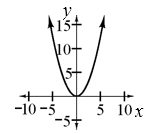### Home > A2C > Chapter 1 > Lesson 1.1.2 > Problem1-21

1-21.

Make a table and graph the function $f\left(x\right)=\frac{1}{2}x^2$. Describe all of the possible input and output values.

Notice that it is a quadratic equation. (Your graph should be a parabola.) Substitute values for $x$ and make a table.

Be sure you include negative numbers in your table.

$\left. \begin{array} { c | c | c | c | c | c } x & { - 4 } & { - 2 } & { 0 } & { 2 } & { 4 } \\ \hline f ( x ) & { 8 } & { 2 } & { 0 } & { 2 } & { 8 } \\ \end{array} \right.$All real numbers are possible for input values and all numbers greater than or equal to zero are possible for output values.

Add points into the eTool table below.
Click the link at the right for the full eTool version: A2C 1-21 HW eTool